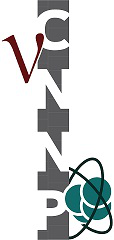#Conference on Neutrino and Nuclear Physics (CNNP2020) Arabella Hotel and Spa, South Africa, 24-28 February 2020

23-28 February 2020
Africa/Johannesburg timezone

## Neutrino-induced reactions on $^{13}$C and $^{16}$O at supernova neutrino energies

28 Feb 2020, 09:40
20m
Main Venue

### Speaker

Toshio Suzuki (Nihon University)

### Description

Neutrino-nucleus reactions on $^{13}$C and $^{16}$O at supernova (SN) energies are investigated by shell-model calculations with the use of new Hamiltonian, which can describe spin responses of nuclei quite well. Cabon-based scintillators and water-Cerenkov scintillators relevent to SN observation and experiments at the spallation neutron sources are now available. Cross sections for various particle and $\gamma$ emission channels are evaluated by the statistical Hauser-Feshbach method.
For $^{13}$C, total reaction cross sections at reactor and solar neutrino energies were studied . Here, we extend our study to SN neutrino energies up to $\approx$50 MeV, and evaluations of partial cross sections for proton and neutron emission channels within the Standard Model . Among them, a reaction channel $^{13}$C ($\bar{\nu}$, $\bar{\nu}$'n) $^{12}$C (2$^{+}$, 4.44 MeV) followed by prompt 4.44 MeV $\gamma$ emission is discussed in relation to the shape distortion in the 5-7 MeV range in the measured neutrino spectrum in the short-baseline reactor neutrino experiments . The cross section is too small to explain the extra enhancement in the spectrum.
Coherent elastic scattering cross section is obtained for ${13}$C, and compared with that for $^{12}$C. Nuclear structure effects in the cross sections are pointed out . Possible merit of large recoil momenta in light nuclei for the study of neutron distributions in nuclei is discussed.
For $^{16}$O, spin-dipole strength, which are the dominant contributions to the cross sections, and neutrino-induced reaction cross sections on $^{16}$O are investigated . Charged-current cross sections induced by SN neutrinos and their dependence on Mikheyev-Smirnov-Wolfenstein neutrino oscillations are discussed for a future SN burst .

 T. Suzuki, A. B. Balantekin and T. Kajino, Phys. Rev. C 86, 015502 (2012).
 T. Suzuki, A. B. Balantekin, T. Kajino and S. Chiba, J. Phys. G 46, 075103 (2019).
 J. M. Berryman, V. Brdar and P. Huber, Phys. Rev. D 99, 055045 (2019).
 T. Suzuki, S. Chiba, T. Yoshida, K. Takahashi and H. Umeda, Phys. Rev. C 98, 034613 (2018).
 K. Nakazato, T. Suzuki and M. Sakuda, PTEP 2018, 123E02 (2018).

### Primary authors

Toshio Suzuki (Nihon University) Prof. A. Baha Balantekin (Wisconsin University) Prof. Toshitaka Kajino (Beihan University & The University of Tokyo) Prof. Satoshi Chiba (Tokyo Institute of Technology)